# Practical Monitoring for tabular data practices ML-OPS Guide Series — 3 – Towards AI — The World’s Leading AI and Technology Publication

#### Author(s): Rashmi Margani

Originally published on Towards AI the World’s Leading AI and Technology News and Media Company. If you are building an AI-related product or service, we invite you to consider becoming an AI sponsor. At Towards AI, we help scale AI and technology startups. Let us help you unleash your technology to the masses.

### Practical Monitoring for tabular data practices ML-OPS Guide Series — 3

Concept drift, data drift & monitoring

In the previous blog as part of the ML-Ops Guide Series, we have discussed what is concept drift, data drift & the importance of monitoring as part of the ML life cycle. Now will see how to remove drift from the training and test data practically for the tabular dataset.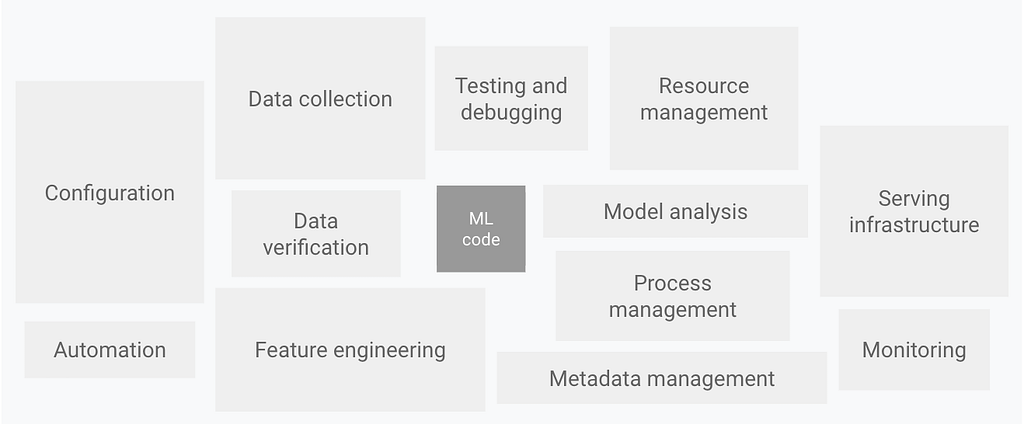Credit:Google cloud

Most of the time in order to detect the drift in the dataset comparison requires a reference distribution which contains fixed data distribution that we compare the production data distribution to. For example, this could be the first month of the training data or the entire training dataset. It depends on the context and the timeframe in which you are trying to detect drift. But obviously, the reference distribution should contain enough samples to represent the training dataset.

For example: To keep it simple, let’s say we have a classification/regression model with 20 features. Let’s say Feature A and Feature B are some of the top contributing features of the model. For this post let’s look at how we can go about trying to see whether features have data drift happening.

To do this will calculate some of the different Statistical(normality test) techniques on the dataset and will discuss going forward,

import pandas as pd
import numpy as np
df=df[[‘Date’,’Close’]]

Here file contains data on Microsoft stock price for 53 weeks. Now, we want to work with returns on the stock, not the price itself, so we will need to do a little bit of data manipulation

df[‘diff’] = pd.Series(np.diff(df[‘Close’]))
df[‘return’] = df[‘diff’]/df[‘Close’]
df = df[[‘Date’, ‘return’]].dropna()
Date return
0 2018-01-01 0.015988
1 2018-01-08 0.004464
2 2018-01-15 0.045111

To make data more sensable, and particularly why we have converted prices to returns which we then want to test for normality using Python, let’s visualize the data using a histogram

from matplotlib import pyplot as plt

plt.hist(df[‘return’])
plt.show()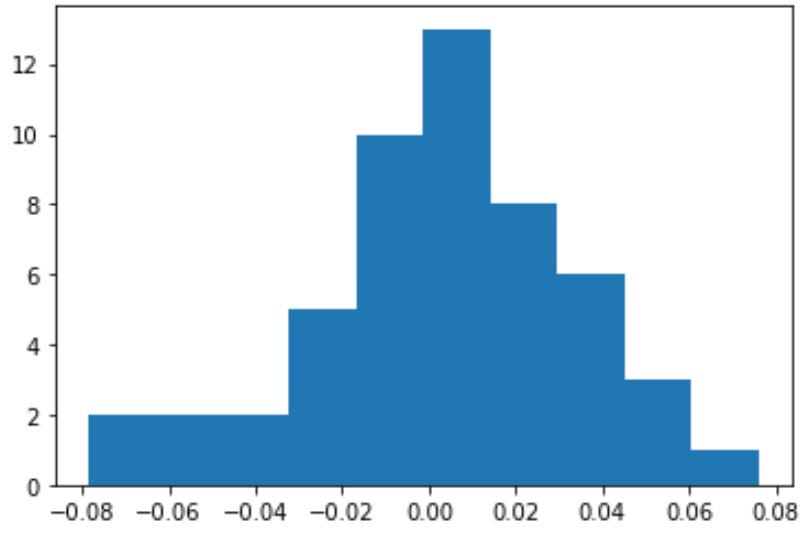If you see the distribution of data visually looks somewhat like the normal distribution. But is it really? That is the question we are going to answer using different statistical methods,

### Q-Q plot Testing

We will start with one of the more visual and less mathematical approaches, quantile-quantile plot.

What is a quantile-quantile plot? It is a plot that shows the distribution of a given data against normal distribution, namely existing quantiles vs normal theoretical quantiles.

Let’s create the Q-Q plot for our data using Python and then interpret the results.

import pylab
import scipy.stats as stats
stats.probplot(df[‘return’], dist=”norm”, plot=pylab)
pylab.show()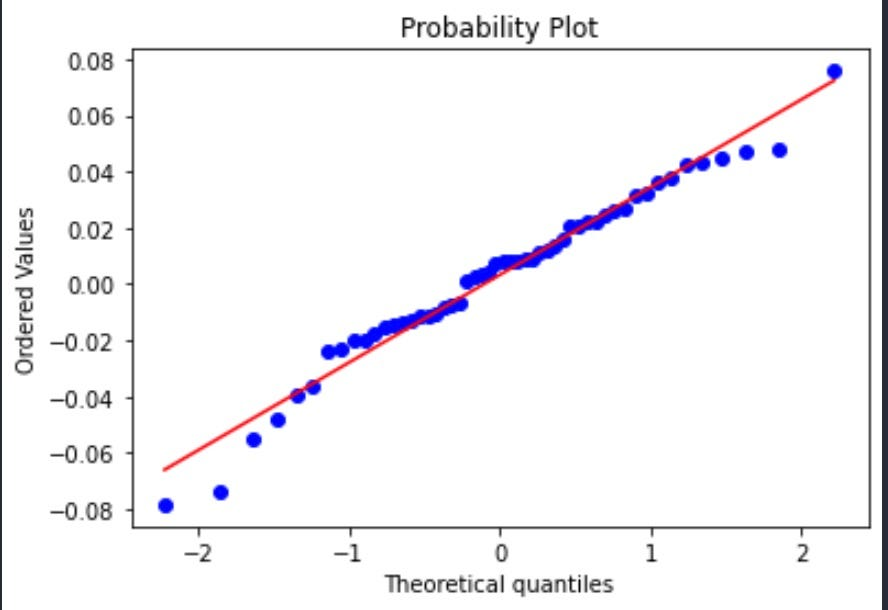Looking at the graph above, we see an upward-sloping linear relationship. For a normal distribution, the observations should all occur on the 45-degree straight line. Do we see such a relationship above? We do partially. So what this can signal to us is that the distribution we are working with is not perfectly normal but close to it.

### Jarque–Bera test

Jarque-Bera is one of the normality tests or specifically the goodness of fit test of matching skewness and kurtosis to that of a normal distribution.

Its statistic is non-negative and large values signal significant deviation from the normal distribution.

The test statistic JB of Jarque-Bera is defined by: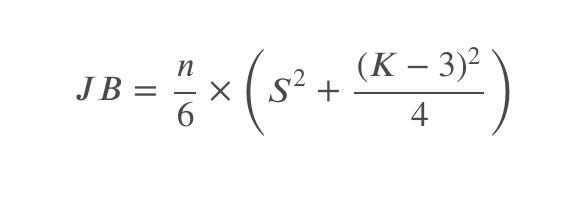where 𝑆 is the sample skewness, 𝐾 is the sample kurtosis, and 𝑛 is the sample size.

The hypotheses:

𝐻0:sample 𝑆 and sample 𝐾 is not significantly different from a normal distribution

𝐻1:sample 𝑆 and sample 𝐾 is significantly different from the normal distribution

Now we can calculate the Jarque-Bera test statistic and find the corresponding 𝑝-value:

from scipy.stats import jarque_bera
result = (jarque_bera(df[‘return’]))
print(f”JB statistic: {result}”)
print(f”p-value: {result}”)
JB statistic: 1.9374105196180924
p-value: 0.37957417002404925

Looking at these results, we fail to reject the null hypothesis and conclude that the sample data follows the normal distribution.

Note: Jarque-Bera test works properly in large samples (usually larger than 2000 observations) at its statistic has a Chi-squared distribution with 2 degrees of freedom)

### Kolmogorov-Smirnov test

One of the most frequent tests for normality is the Kolmogorov-Smirnov test (or K-S test). A major advantage compared to other tests is that the Kolmogorov-Smirnov test is nonparametric, meaning that it is distribution-free.

Here we focus on the one-sample Kolmogorov-Smirnov test because we are looking to compare a one-dimensional probability distribution with a theoretically specified distribution (in our case it is normal distribution).

The Kolmogorov-Smirnov test statistic measures the distance between the empirical distribution function (ECDF) of the sample and the cumulative distribution function of the reference distribution.

In our example, the empirical distribution function will come from the data on returns we have compiled earlier. And since we are comparing it to the normal distribution, we will work with the cumulative distribution function of the normal distribution.

So far this sounds very technical, so let’s try to break it down and visualize it for better understanding.

Step 1:

Let’s create an array of values from a normal distribution with a mean and standard deviation of our returns data:

data_norm = np.random.normal(np.mean(df[‘return’]), np.std(df[‘return’]), len(df))

Using np.random.normal() we created the data_norm which is an array that has the same number of observations as df[‘return’] and also has the same mean and standard deviation.

The intuition here would be that if we assume some parameters of the distribution (mean and standard deviation), what would be the numbers with such parameters that would form a normal distribution.

Step 2:

Next, what we are going to do is use np.histogram() on both datasets to sort them and allocate them to bins:

values, base = np.histogram(df[‘return’])
values_norm, base_norm = np.histogram(data_norm)

Note: by default, the function will use bins=10, which you can adjust based on the data you are working with.

Step 3:

Use np.cumsum() to calculate cumulative sums of arrays created above:

cumulative = np.cumsum(values)
cumulative_norm = np.cumsum(values_norm)

Step 4:

Plot the cumulative distribution functions:

plt.plot(base[:-1], cumulative, c=’blue’)
plt.plot(base_norm[:-1], cumulative_norm, c=’green’)
plt.show()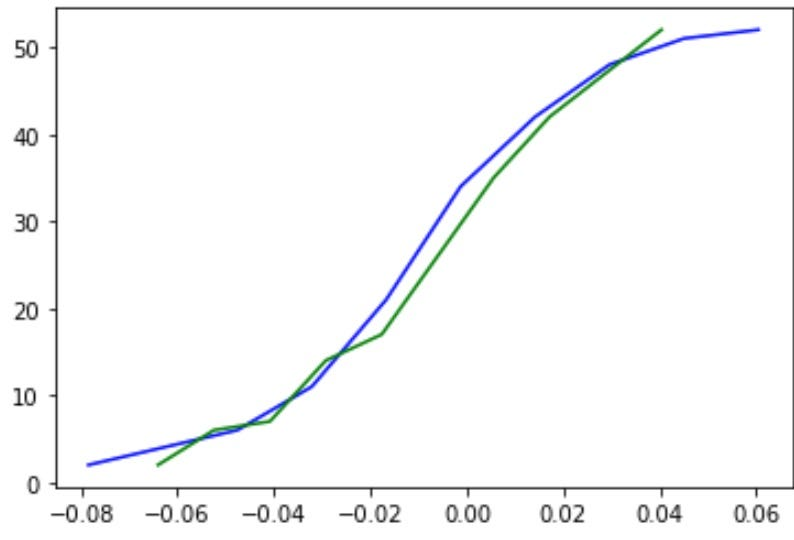Where the blue line is the ECDF (empirical cumulative distribution function) of df[‘return’], and the green line is the CDF of a normal distribution.

Step 4 Alternative:

You can create the graph quicker by using seaborn and only need df[‘return’] and data_norm from Step 1:

import seaborn as sns
sns.ecdfplot(df[‘return’], c=’blue’)
sns.ecdfplot(data_norm, c=’green’)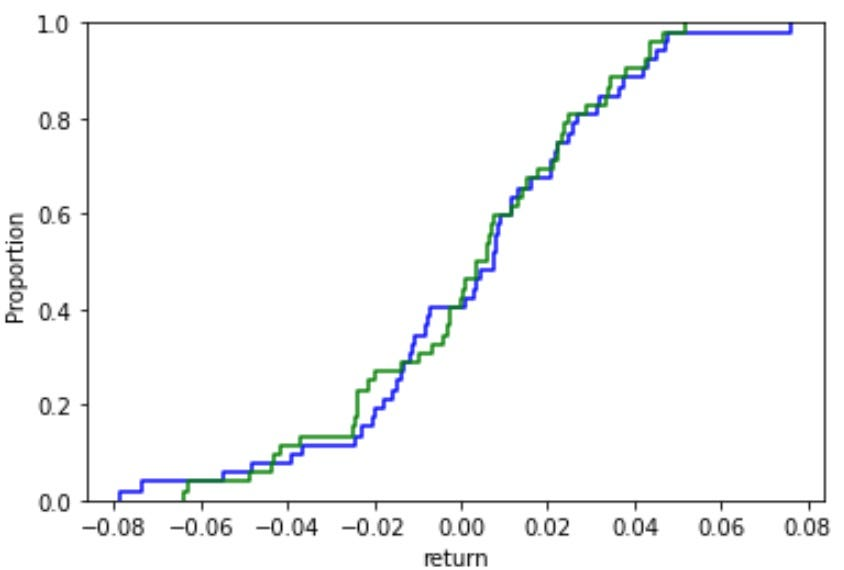Now, Let’s go back to the Kolmogorov-Smirnov test now after visualizing these two cumulative distribution functions. Kolmogorov-Smirnov test is based on the maximum distance between these two curves (blue-green) with the following hypotheses:

𝐻0: two samples are from the same distribution

𝐻1: two samples are from different distributions

We define ECDF as: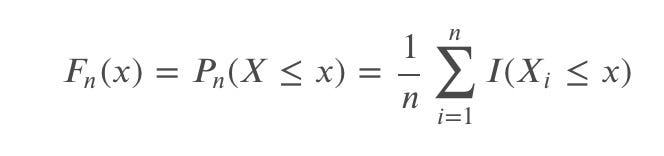which counts the proportion of the sample observations below level 𝑥.

We define a given (theoretical) CDF as: 𝐹(𝑥). In the case of testing for normality, 𝐹(𝑥) is the CDF of a normal distribution.

The Kolmogorov-Smirnov statistic is defined as: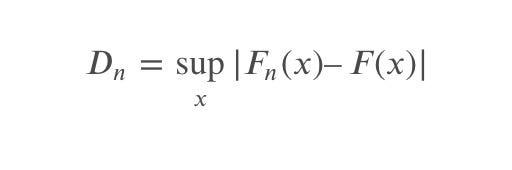Intuitively, this statistic measures the largest absolute distance between two distribution functions for all 𝑥 values.

Using the graph above, here is my estimated supremum:Here blue line indicates supremum. Calculating the value of 𝐷𝑛 and comparing it with the critical value (assume 5%) of 𝐷0.05, we can either reject or fail to reject the null hypothesis.

Back to our example, let’s perform the K-S test for the Microsoft stock returns data:

from scipy.stats import kstest
result = (kstest(df[‘return’], cdf=’norm’))
print(f”K-S statistic: {result}”)
print(f”p-value: {result}”)
K-S statistic: 0.46976096086398267
p-value: 4.788934452701707e-11

Since the 𝑝-value is significantly less than 0.05, we reject the null hypothesis and accept the alternative hypothesis that the two samples tested are not from the same cumulative distribution, meaning that the returns on Microsoft stock are not normally distributed.

### Anderson-Darling test

Anderson-Darling test (A-D test) is a modification of the Kolmogorov-Smirnov test described above. It tests whether a given sample of observations is drawn from a given probability distribution (in our case from a normal distribution).

𝐻0: the data comes from a specified distribution

𝐻1: the data doesn’t come from a specified distribution

The A-D test is more powerful than the K-S test since it considers all of the values in the data and not just the one that produces maximum distance (like in the K-S test). It also assigns more weight to the tails of a fitted distribution.

This test belongs to the quadratic empirical distribution function (EDF) statistics and is given by: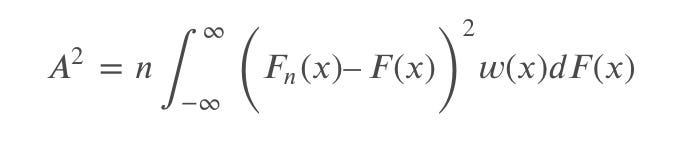where 𝐹 is the hypothesized distribution (in our case, normal distribution), 𝐹𝑛 is the ECDF (calculations discussed in the previous section), and 𝑤(𝑥) is the weighting function.

The weighting function is given by: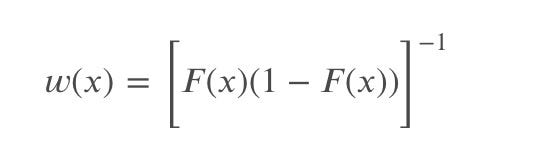which allows placing more weight on observations in the tails of the distribution.

Given such a weighting function, the test statistic can be simplified to: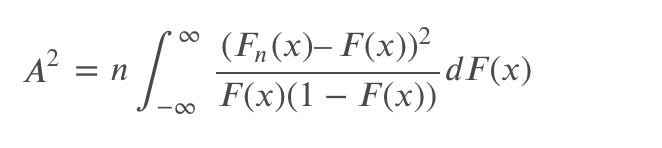Suppose we have a sample of data 𝑋 and we want to test whether this sample comes from a cumulative distribution function (𝐹(𝑥)) of the normal distribution.

We need to sort the data such that 𝑥1<𝑥2<…<𝑥𝑛 and then compute the 𝐴2 statistic as: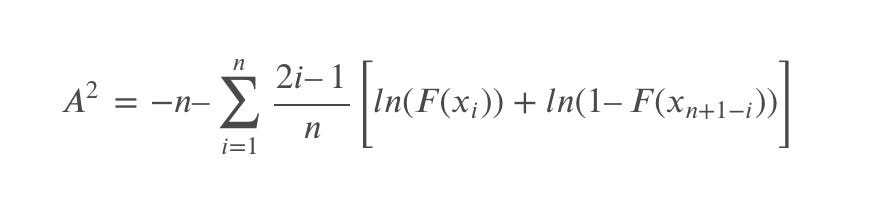Back to our example, let’s perform the A-D test in Python for the Microsoft stock returns data:

from scipy.stats import anderson
result = (anderson(df[‘return’], dist=’norm’))
print(f”A-D statistic: {result}”)
print(f”Critical values: {result}”)
print(f”Significance levels: {result}”)
A-D statistic: 0.3693823006816217
Critical values: [0.539 0.614 0.737 0.86 1.023]
Significance levels: [15. 10. 5. 2.5 1. ]

The first row of the output is the A-D test statistic, which is around 0.37; the third row of the output is a list with different significance levels (from 15% to 1%); the second row of the output is a list of critical values for the corresponding significance levels.

Let’s say we want to test our hypothesis at the 5% level, meaning that the critical value we will use is 0.737 (from the output above). Since the computer A-D test statistic (0.37) is less than the critical value (0.737), we fail to reject the null hypothesis and conclude that the sample data of Microsoft stock returns come from a normal distribution.

### Shapiro-Wilk test

Shapiro-Wilk test (S-W test) is another test for normality in statistics with the following hypotheses:

𝐻0: distribution of the sample is not significantly different from a normal distribution

𝐻1: distribution of the sample is significantly different from a normal distribution

Unlike the Kolmogorov-Smirnov test and the Anderson-Darling test, it doesn’t base its statistic calculation on ECDF and CDF, rather it uses constants generated from moments from a normally distributed sample.

The Shapiro-Wilk test statistics is defined as: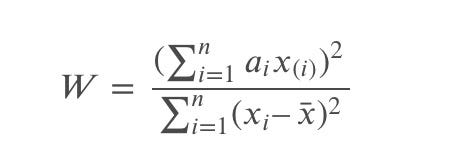where 𝑥(𝑖) is the 𝑖th smallest number in the sample (𝑥1<𝑥2<…<𝑥𝑛); and 𝑎𝑖 are constants generated from var, cov, mean from a normally distributed sample.

Back to our example, let’s perform the S-W test in Python for the Microsoft stock returns data:

from scipy.stats import shapiro

result = (shapiro(df[‘return’]))

print(f”S-W statistic: {result}”)
print(f”p-value: {result}”)

S-W statistic: 0.9772366881370544
p-value: 0.41611215472221375

Given the large 𝑝-value (0.42) which is greater than 0.05 (>0.05), we fail to reject the null hypothesis and conclude that the sample is not significantly different from a normal distribution.

Note: one of the biggest limitations of this test is the size bias, meaning that the larger the size of the sample is, the more likely you are to get a statistically significant result.

And to download the notebook and dataset please check Github

Note: data drift and concept drift are vast topics to cover where a new book can be written. So some other useful tools to explore as a reference to having some more breadth and depth for the various flavor of the dataset such as TorchDrift, EvidentlyAI, Alibi detect, River.

On next series will look into different aspects of implementing an impactful data pipeline which is crucial as part of the ML system development phase.Practical Monitoring for tabular data practices ML-OPS Guide Series — 3 was originally published in Towards AI on Medium, where people are continuing the conversation by highlighting and responding to this story.

Published via Towards AI